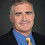## Monday, February 17, 2003

### Complexity Class of the Week: BPPpath

Previous CCW

Let us call a nondeterministic Turing machine M balanced if for every input x, all of its computational paths have the same length, i.e., the number of nondeterministic bits depends only on x and not on previous guesses. We can define the characterize class BPP as follows:

L is in BPP if there is a balanced nondeterministic polynomial-time M such that

1. If x is in L then there are at least twice as many accepting as rejecting paths of M(x).
2. If x is not in L then there are at least twice as many rejecting as accepting paths of M(x).
Suppose we use the same definition without the "balanced" requirement. This gives us the class BPPpath studied mostly in a 1997 paper of Han, Hemaspaandra and Thierauf.

BPPpath contains BPP by definition but it contains much more. Let us show that SAT is contained in BPPpath.

Let φ be a formula with n variables. Consider the following machine M(φ): Guess an assignment a for φ. If a is rejecting then reject. If a is accepting then guess n+1 bits, ignore them and accept.

If φ is not satisfiable then M(φ) will only have rejecting paths. If φ is satisfiable then it will have at least 2n+1 accepting paths and at most 2n-1 rejecting paths fulfilling the requirements of the definition of BPPpath.

Han, Hemaspaandra and Thierauf prove many other results about BPPpath. The class contains MA and PNP[log] (P with O(log n) queries to an NP language like SAT). BPPpath is contained in PΣ2[log], BPPNP and PP.

Whether BPPpath is contained in ZPPNP or Σ2 remain the most interesting open questions about this class.

1.Are there relativisations for the "BPPpath in ZPPNP or Σ2"? (I do not know if I will get an answer on a four-year-old post :) )

2.Yes there is a relatized world where BPPpath is not contained in Σ2 (and thus also not contained in ZPP^NP). See this post.

3.Is there BPLpath? If PL=NL=UL=ParityL we would think PL=NL=UL=ParityL=BPLpath. Would PL=RL follow?

4.Is there a consequence if BPLpath=PL? We know PL=PLP by Jung's theorem. Can Jung's theorem be improvable to BPL=BPLP if BPLpath=PL or BPLpath=PP?